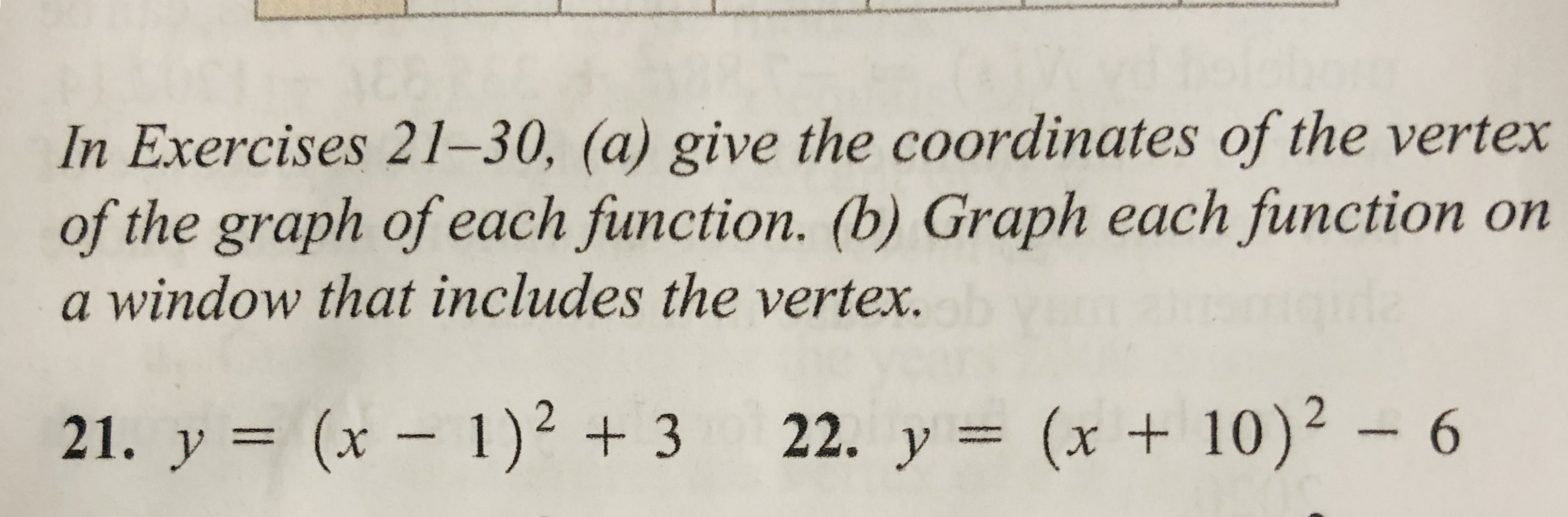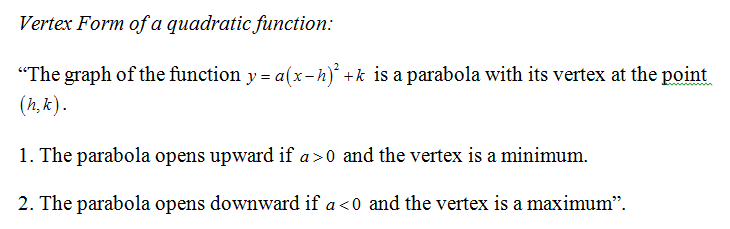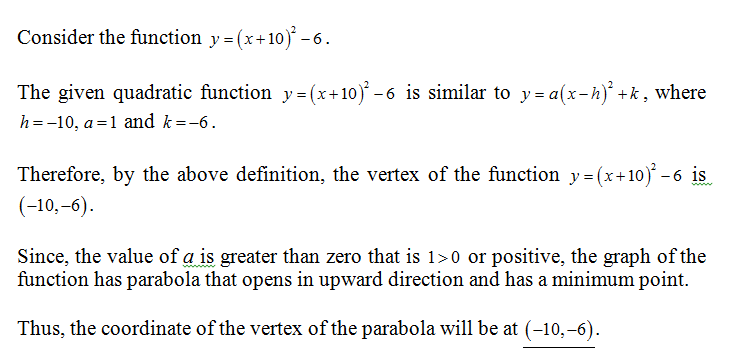# In Exercises 21–30, (a) give the coordinates of the vertexof the graph of each function. (b) Graph each function ona window that includes the vertex.gida(x +10)²21. y = (x – 1)² + 3 22. y = (x + 10)² – 6

Question
52 views

Question #22help_outlineImage TranscriptioncloseIn Exercises 21–30, (a) give the coordinates of the vertex of the graph of each function. (b) Graph each function on a window that includes the vertex. gida (x +10)² 21. y = (x – 1)² + 3 22. y = (x + 10)² – 6 fullscreen
check_circle

star
star
star
star
star
1 Rating
(a) Definition used:Calculation:...

### Want to see the full answer?

See Solution

#### Want to see this answer and more?

Solutions are written by subject experts who are available 24/7. Questions are typically answered within 1 hour.*

See Solution
*Response times may vary by subject and question.
Tagged in